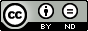### Probability Functions of Order Statistics from Discrete Uniform Distribution

Ayse Metin Karakaş, S. Çalik

#### Abstract

In this paper, we firstly give basic definitions and theorems for order statistics. Later, we show that r. probability function of order statistics from discrete uniform distribution can be obtained in another form.

#### Keywords

Order statistics, Distribution function, Discrete Uniform Distribution, Moments, Probability Function

PDF

#### References

Khatri, C. G. (1962). Distributions of order statistics for discrete case. Annals of the Institute of Statistical Mathematics, 14(1), 167-171.

Das Gupta, S. & Sarkar, S.K. (1984). On TP2 and log-concavity. In Tong, Y.L. (ed.), Inequalities in statistics and probability, London: Institute of Mathematical, pp. 54–58.

Kabe, D. G. (1969). Some distribution problems of order statistics from discrete populations. Annals of the Institute of Statistical Mathematics, 21(1), 551-556.

David, H. A., & Nagaraja, H. N. (1981). Order statistics. New York: John Willey & Sons. David2Order Statistics1981.

Nagaraja, H. N. (1982). Some nondegenerate limit laws for the selection differential. Annals of Statistics, 10, 1306-1310.

Arnold, B. C., Balakrishnan, N., & Nagaraja, H. N. (1992). A first course in order statistics (Vol. 54). Siam.

Ahsanullah, M., & Nevzorov, V. B. (2001). Ordered Random Variables, Nova Sci. Publ., Huntington, NY, 200(1).

Çalik, S., & Güngör, M. (2004). On the expected values of the sample maximum of order statistics from a discrete uniform distribution. Applied mathematics and computation, 157(3), 695-700.

Turan, A. (2008) Kesikli Düzgün Dağılımdaki Sıra İstatistiklerin Örnek Ekstremlerinin Mometleri, Fırat University, Science Institute, Elazığ.

Calik, S., et al., On The Moments of Order Statistics from Discrete Distributions, Pak. J. Statist., 26 (2010), 2, pp. 417-426.

Buğatekin Turan, A.,. Generalized Moments of Sample Extremes of Order Statistics from Discrete Uniform Distribution, Fırat University, Institute of Science 2011.

Calik, S., & Bugatekin, A. T. (2018). Moments of Sample Extremes of Order Statistics from Discrete Uniforms Dıstrıbutıon and Numerıcal Results. Thermal Science.

DOI: https://doi.org/10.24203/ajfam.v7i1.5668

### Refbacks

• There are currently no refbacks.

Copyright (c) 2019 Asian Journal of Fuzzy and Applied Mathematics